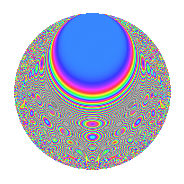# Properties

 Label 1350.2.hLevel 1350 Weight 2 Character orbit h Rep. character $$\chi_{1350}(271,\cdot)$$ Character field $$\Q(\zeta_{5})$$ Dimension 160 Sturm bound 540

# Related objects

## Defining parameters

 Level: $$N$$ = $$1350 = 2 \cdot 3^{3} \cdot 5^{2}$$ Weight: $$k$$ = $$2$$ Character orbit: $$[\chi]$$ = 1350.h (of order $$5$$ and degree $$4$$) Character conductor: $$\operatorname{cond}(\chi)$$ = $$25$$ Character field: $$\Q(\zeta_{5})$$ Sturm bound: $$540$$

## Dimensions

The following table gives the dimensions of various subspaces of $$M_{2}(1350, [\chi])$$.

Total New Old
Modular forms 1128 160 968
Cusp forms 1032 160 872
Eisenstein series 96 0 96

## Trace form

 $$160q - 40q^{4} - 4q^{7} + O(q^{10})$$ $$160q - 40q^{4} - 4q^{7} + 6q^{10} + 8q^{13} - 40q^{16} + 12q^{19} + 8q^{22} + 18q^{25} + 6q^{28} + 6q^{31} - 24q^{34} - 32q^{37} + 6q^{40} + 56q^{43} + 12q^{46} + 220q^{49} + 8q^{52} + 30q^{55} + 48q^{58} + 40q^{61} - 40q^{64} - 46q^{70} + 124q^{73} - 8q^{76} + 56q^{79} + 80q^{82} - 160q^{85} - 2q^{88} + 20q^{91} - 16q^{94} - 18q^{97} + O(q^{100})$$

## Decomposition of $$S_{2}^{\mathrm{new}}(1350, [\chi])$$ into newform subspaces

The newforms in this space have not yet been added to the LMFDB.

## Decomposition of $$S_{2}^{\mathrm{old}}(1350, [\chi])$$ into lower level spaces

$$S_{2}^{\mathrm{old}}(1350, [\chi]) \cong$$ $$S_{2}^{\mathrm{new}}(25, [\chi])$$$$^{\oplus 8}$$$$\oplus$$$$S_{2}^{\mathrm{new}}(50, [\chi])$$$$^{\oplus 4}$$$$\oplus$$$$S_{2}^{\mathrm{new}}(75, [\chi])$$$$^{\oplus 6}$$$$\oplus$$$$S_{2}^{\mathrm{new}}(150, [\chi])$$$$^{\oplus 3}$$$$\oplus$$$$S_{2}^{\mathrm{new}}(225, [\chi])$$$$^{\oplus 4}$$$$\oplus$$$$S_{2}^{\mathrm{new}}(450, [\chi])$$$$^{\oplus 2}$$$$\oplus$$$$S_{2}^{\mathrm{new}}(675, [\chi])$$$$^{\oplus 2}$$

## Hecke Characteristic Polynomials

There are no characteristic polynomials of Hecke operators in the database